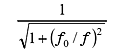FFT analyzer FAQWhat is the frequency characteristic of AC coupling?The AC coupling is the first order high pass filter which consists of the condenser (C) and resistance (R). The main purpose of AC coupling is to cut the low frequency components including the DC components of the input signal. The gain character is as follows.(1)

In above formula, f0 is the cut off frequency of high pass filter and normally it is 0.5 Hz in our FFT analyzer. If the frequency f of the input signal is 0.5 Hz (=f0), the value of formula (1) is around 0.707 (-3 dB) and when the frequency f is 1 Hz (=f0), the value of formula (1) is around 0.89 (-0.97 dB).

Revised:2009/11/16